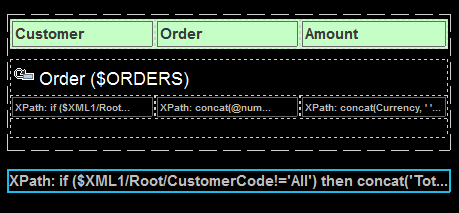# Altova MobileTogether Designer

 Sub Page: Decimal Totals in XPath Scroll Home Prev Top Next More

To complete the design, we will add a label that displays the total amount of the displayed orders. Do this as follows:

1.Drag a Label control from the Controls Pane and drop it below the Orders table (see screenshot below).

2.In the Styles & Properties Pane, Click the XPath icon of the control's Text property.

3.In the In the Edit XPath/XQuery Expression dialog that appears, enter the XPath expression to calculate the total amounts (the expression is given below), and click OK.## The XPath expression to calculate the total amount

We need to calculate totals in two events: (i) for the orders of the selected customer, and (ii) for all orders. This can be done with the following XPath expression:

if (\$XML1/Root/CustomerCode!='All')

then concat('Total: 'xs:decimal(sum (\$ORDERS//Order[CustomerCode=\$XML1/Root/CustomerCode]/OrderAmount)))

else concat('Total: 'xs:decimal(sum (\$ORDERS//OrderAmount)))

This XPath expression works as follows:

1.The if clause of the expression tests whether the element \$XML1/Root/CustomerCode contains the string All.

2.If the element \$XML1/Root/CustomerCode does not contain the string All, then the OrderAmount element of all Order elements that have their CustomerCode element content equal to the content of the \$XML1/Root/CustomerCode element will be selected. These will be the amounts of those Order elements of the customer that was selected by the end user. Remember that the customer's CustomerCode has been stored in the \$XML1 page source (see Top Page: Action Group, Go to Sub Page).

3.If the element \$XML1/Root/CustomerCode contains the string All, then all OrderAmount elements will be selected.

The selected OrderAmount elements are summed using the sum() function of XPath. Since the sum() function uses the xs:double type and returns an xs:double number, the amount will have more than the two decimal places we require in a currency. We therefore use the xs:decimal type converter to round the xs:double to a two-decimal place number.